Next: 3.3.1 First Order in Up: 3. Many-Mody Multi-Scale Method Previous: 3.2.4 Ansatz

# 3.3 Approximations to the vertex

In this section we provide the remaining details of the-approximation. Previous studies, as well as our own results, have shown that in the positiveHubbard model the long-ranged contributions to the self-energy are dominated by the spin and charge fluctuations of the system. In contrast, pairing fluctuations are less significant and do not change the qualitative multi-scale results, unless very low temperatures are considered. The self-energy determination in the results section for the lowest order inapproximation toconsiders all three channels. However, the remaining higher order cases are restricted to only the self-energy contribution of the particle-hole channel. An equivalent derivation to the following can be trivially extended to the particle-particle channel.

Starting with the-approximation introduced in sections 3.2.2 (Eqs. 3.8 and 3.9), the Bethe-Salpeter equation (Eq. 3.7) for the approximated large cluster reducible vertex function can be coarse-grained to the small cluster (see section 3.6 for further details):(3.14)

where we coarse grained the bare particle-hole susceptibility bubble (internal legs in Fig. 3.4 which are lattice Green's functions). We call this coarse grained susceptibility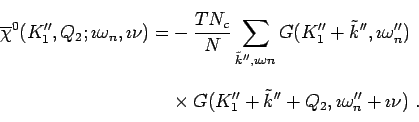(3.15)

Furthermore, while the coarse-graining (sum over internal moment) is to the small cluster, the self-energy inoriginates from the large cluster. The resulting reducible vertex functionhowever, is still only defined for small cluster momenta but incorporates large cluster corrections from the coarse grained susceptibility. Due to these long-ranged contributions, the reducible vertex constructed in this manner is not equivalent to a QMC evaluated small cluster.

The resulting Bethe-Salpeter equation (3.14) is most easily solved forin the following matrix form(3.16)

where the matrix indices correspond to the internal momenta and frequency.

At this point, the self-energies can now finally be evaluated using the Dyson equation as illustrated in Fig. 3.3. On the large cluster this yields: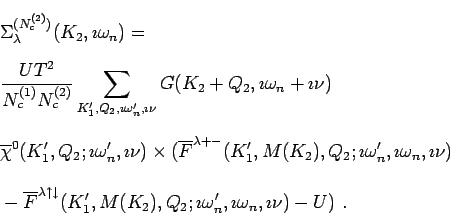(3.17)

Here we interpolate the small cluster momentum(for details see section 3.6) and subtractedin the parenthesis to prevent an over counting of the second order term.

In the calculation of the transverse spin fluctuation part, recall thatwhere we defineas the correlation function of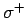and, andas the correlation function formed from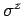. Then as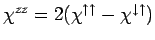, we have that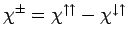and. This means that for the self-energy on the large cluster,(3.18)

While for the real-space implementation of the Ansatz, knowledge of the large cluster self-energy in the-approximation is sufficient, the momentum-space version requires the corresponding self-energy diagrams on the small cluster as well. An equivalent calculation on the small cluster yields for the self-energy in-approximation(3.19)

where all single-particle propagators have been replaced by, including the ones entering the bare bubble.

We want to reiterate that the-approximated self energy thus obtained, is only accurate for long-ranged correlations. In order to obtain a viable multi-scale solution, the neglected short-ranged correlations have to be accounted for by means of the Ansatz, as outlined in the previous section.

SubsectionsNext: 3.3.1 First Order in Up: 3. Many-Mody Multi-Scale Method Previous: 3.2.4 Ansatz
© Cyrill Slezak